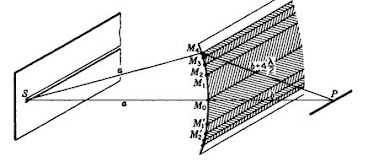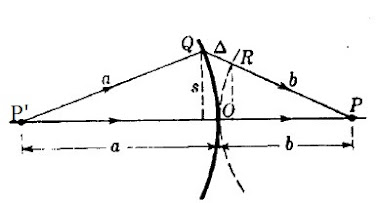## Tuesday, May 3, 2022

### A First Look At Fresnel Diffraction (Part 1)

Fresnel diffraction is the less well known version of diffraction and this is on account of it being more mathematically demanding than Fraunhofer diffraction - which I've already covered in several instalments, e.g.

The Basics of Fraunhofer Diffraction & Its Applications (Pt. 1) (brane-space.blogspot.com)

We begin our introduction by noting the irony of this form, i.e. it is the easiest to observe but also requires much more complex mathematical theory to explain it. The latter aspect is because Fraunhofer diffraction - dealing with light sources at a distance - treats plane waves, while Fresnel diffraction treats spherical waves, wavefronts.Spherical wavefronts in Fresnel diffraction

By their very nature then - because of the geometry - one will enter a more difficult mathematical realm.  Fortunately, because Fresnel diffraction was the first type to be observed, there was a longer lead time to develop the math behind it.

How is it that Fresnel is easier to observe?  Well, for one thing,  one doesn't need lenses but can observe effects directly, such as shadows produced around edges. For example, consider the following: An opaque object - say coin - placed in the path of a point light source will produce a shadow with a fairly well defined outline with the same shape as the object. (The edge is not absolutely sharp when observed closely, however, but it seen to have alternating dark and light bands in the immediate vicinity of the edge.  According to Fresnel, this is because in the regions beyond the limits of the geometrical shadow secondary wavelets arrive with phase relations that destructively interfere with each other and produce practically complete darkness.  In other regions where there is slight reinforcement we observe lighter bands of shadow.

Central to Fresnel's approach to most diffraction problems are his "half period zones" whereby he found that a slightly divergent spherical wave will produce a point ahead of the wave and the resulting paths could be quantified in terms of the wavelength l, e.g.

Here we have a spherical wave segment BCDE of monochromatic light traveling to the right to point P. Each point on the spherical segment can be thought of as an origin for secondary wavelets for which the resultant is found to terminate at point P.  The problem then becomes how to find the resultant effect.  Fresnel's approach prescribed dividing the wave front into a series of concentric "zones" as are visible in the image as something like circles on a target.

The specific technique then means drawing a series of circles around the point O.  The distances from point O, measured along the arc are: s 1 ,  s 2 ,   s 3,  ..... s n.  They are such that each successive circle is a half-wavelength farther from P.  Therefore, if the distance OP = b, we would have: b  + l /2,  b  + 2l /2,  b  + 3l /2,  ........b  +   m l /2  and so on.

The areas Am   of the  rings (zones) between successive circles are roughly equal to each other which can be proven by reference to the diagram below:Here a section of the wave spreads out from P' with radius a.  If a circle of radius b is now drawn with center at P and tangent to the wave front at its pole O, the path P' QP is longer than the path POP by the short segmented denoted by D .  Then, quantifying:

D  =  s 2 / 2a   +  2 / 2b     =   2  [(a + b)/ 2 ab]

Then the radii  sm   of the Fresnel zones are such that:

l /2   =    s m 2  [(a + b)/ 2 ab]

Then the area of any one zone is expressed:

A m(D) = p  (s m   -  s m-1  2  =    l /2 {2 ab /  (a + b)} =

l ab/ (a + b)

To the approximation considered, the area   is therefore independent of m .  (A more exact computation would disclose that the area icreases only very slowly with m.)

Suggested Problems:

1) The innermost zone of a zone plate has a diameter 0.425mm.

(a)Find the focal length of the plate when it is used with parallel incident light of wavelength 4471 Å from a helium lamp.

(b) Find the first subsidiary focal length for the zone plate.

2) A zone plate is to be set up on an optical bench where it will be used as an enlarging lens.  Its innermost zone will have a diameter of 0.2250 mm with monochromatic green light of wavelength 4800 Å  to be used.  If all enlargements are to be eightfold in diameter find the focal length f of the zone plate.